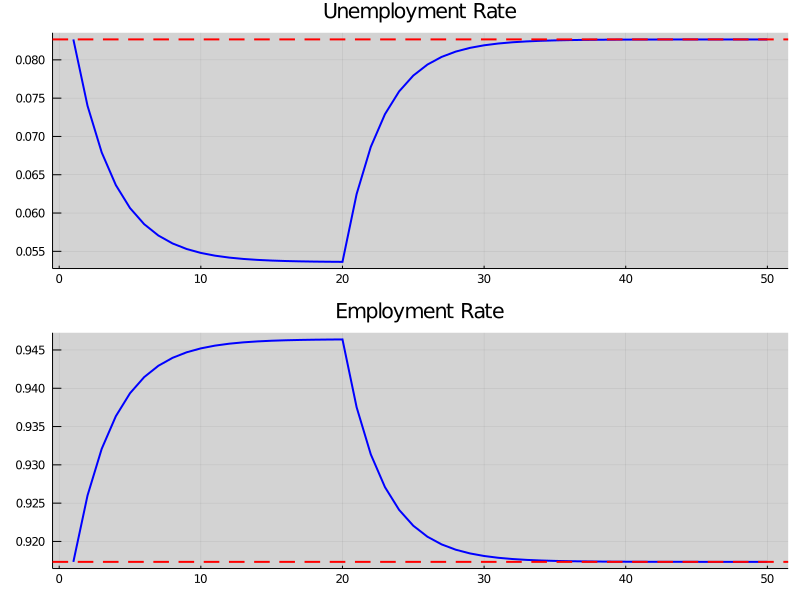# A Lake Model of Employment and Unemployment¶

## Overview¶

This lecture describes what has come to be called a lake model.

The lake model is a basic tool for modeling unemployment.

It allows us to analyze

• flows between unemployment and employment
• how these flows influence steady state employment and unemployment rates

It is a good model for interpreting monthly labor department reports on gross and net jobs created and jobs destroyed.

The “lakes” in the model are the pools of employed and unemployed.

The “flows” between the lakes are caused by

• firing and hiring
• entry and exit from the labor force

For the first part of this lecture, the parameters governing transitions into and out of unemployment and employment are exogenous.

Later, we’ll determine some of these transition rates endogenously using the McCall search model.

We’ll also use some nifty concepts like ergodicity, which provides a fundamental link between cross-sectional and long run time series distributions.

These concepts will help us build an equilibrium model of ex ante homogeneous workers whose different luck generates variations in their ex post experiences.

### Prerequisites¶

Before working through what follows, we recommend you read the lecture on finite Markov chains.

You will also need some basic linear algebra and probability.

## The Model¶

The economy is inhabited by a very large number of ex ante identical workers.

The workers live forever, spending their lives moving between unemployment and employment.

Their rates of transition between employment and unemployment are governed by the following parameters:

• $\lambda$, the job finding rate for currently unemployed workers
• $\alpha$, the dismissal rate for currently employed workers
• $b$, the entry rate into the labor force
• $d$, the exit rate from the labor force

The growth rate of the labor force evidently equals $g=b-d$.

### Aggregate Variables¶

We want to derive the dynamics of the following aggregates

• $E_t$, the total number of employed workers at date $t$
• $U_t$, the total number of unemployed workers at $t$
• $N_t$, the number of workers in the labor force at $t$

We also want to know the values of the following objects

• The employment rate $e_t := E_t/N_t$.
• The unemployment rate $u_t := U_t/N_t$.

(Here and below, capital letters represent stocks and lowercase letters represent flows)

### Laws of Motion for Stock Variables¶

We begin by constructing laws of motion for the aggregate variables $E_t,U_t, N_t$.

Of the mass of workers $E_t$ who are employed at date $t$,

• $(1-d)E_t$ will remain in the labor force
• of these, $(1-\alpha)(1-d)E_t$ will remain employed

Of the mass of workers $U_t$ workers who are currently unemployed,

• $(1-d)U_t$ will remain in the labor force
• of these, $(1-d) \lambda U_t$ will become employed

Therefore, the number of workers who will be employed at date $t+1$ will be

$$E_{t+1} = (1-d)(1-\alpha)E_t + (1-d)\lambda U_t$$

A similar analysis implies

$$U_{t+1} = (1-d)\alpha E_t + (1-d)(1-\lambda)U_t + b (E_t+U_t)$$

The value $b(E_t+U_t)$ is the mass of new workers entering the labor force unemployed.

The total stock of workers $N_t=E_t+U_t$ evolves as

$$N_{t+1} = (1+b-d)N_t = (1+g)N_t$$

Letting $X_t := \left(\begin{matrix}U_t\\E_t\end{matrix}\right)$, the law of motion for $X$ is

$$X_{t+1} = A X_t \quad \text{where} \quad A := \begin{pmatrix} (1-d)(1-\lambda) + b & (1-d)\alpha + b \\ (1-d)\lambda & (1-d)(1-\alpha) \end{pmatrix}$$

This law tells us how total employment and unemployment evolve over time.

### Laws of Motion for Rates¶

Now let’s derive the law of motion for rates.

To get these we can divide both sides of $X_{t+1} = A X_t$ by $N_{t+1}$ to get

$$\begin{pmatrix} U_{t+1}/N_{t+1} \\ E_{t+1}/N_{t+1} \end{pmatrix} = \frac1{1+g} A \begin{pmatrix} U_{t}/N_{t} \\ E_{t}/N_{t} \end{pmatrix}$$

Letting

$$x_t := \left(\begin{matrix} u_t\\ e_t \end{matrix}\right) = \left(\begin{matrix} U_t/N_t\\ E_t/N_t \end{matrix}\right)$$

we can also write this as

$$x_{t+1} = \hat A x_t \quad \text{where} \quad \hat A := \frac{1}{1 + g} A$$

You can check that $e_t + u_t = 1$ implies that $e_{t+1}+u_{t+1} = 1$.

This follows from the fact that the columns of $\hat A$ sum to 1.

## Implementation¶

Let’s code up these equations.

Here’s the code:

### Setup¶

In :
using InstantiateFromURL
# optionally add arguments to force installation: instantiate = true, precompile = true
github_project("QuantEcon/quantecon-notebooks-julia", version = "0.8.0")

In :
using LinearAlgebra, Statistics
using Distributions, Expectations, NLsolve, Parameters, Plots
using QuantEcon, Roots, Random

In :
gr(fmt = :png);

In :
LakeModel = @with_kw (λ = 0.283, α = 0.013, b = 0.0124, d = 0.00822)

function transition_matrices(lm)
@unpack λ, α, b, d = lm
g = b - d
A = [(1 - λ) * (1 - d) + b      (1 - d) * α + b
(1 - d) * λ                 (1 - d) * (1 - α)]
Â = A ./ (1 + g)
return (A = A, Â = Â)
end

function rate_steady_state(lm)
@unpack Â = transition_matrices(lm)
sol = fixedpoint(x -> Â * x, fill(0.5, 2))
converged(sol) || error("Failed to converge in $(result.iterations) iterations") return sol.zero end function simulate_stock_path(lm, X0, T) @unpack A = transition_matrices(lm) X_path = zeros(eltype(X0), 2, T) X = copy(X0) for t in 1:T X_path[:, t] = X X = A * X end return X_path end function simulate_rate_path(lm, x0, T) @unpack Â = transition_matrices(lm) x_path = zeros(eltype(x0), 2, T) x = copy(x0) for t in 1:T x_path[:, t] = x x = Â * x end return x_path end  Out: simulate_rate_path (generic function with 1 method) Let’s observe these matrices for the baseline model In : lm = LakeModel() A, Â = transition_matrices(lm) A  Out: 2×2 Array{Float64,2}: 0.723506 0.0252931 0.280674 0.978887 In : Â  Out: 2×2 Array{Float64,2}: 0.720495 0.0251879 0.279505 0.974812 And a revised model In : lm = LakeModel(α = 2.0) A, Â = transition_matrices(lm) A  Out: 2×2 Array{Float64,2}: 0.723506 1.99596 0.280674 -0.99178 In : Â  Out: 2×2 Array{Float64,2}: 0.720495 1.98765 0.279505 -0.987652 ### Aggregate Dynamics¶ Let’s run a simulation under the default parameters (see above) starting from$ X_0 = (12, 138) $In : lm = LakeModel() N_0 = 150 # population e_0 = 0.92 # initial employment rate u_0 = 1 - e_0 # initial unemployment rate T = 50 # simulation length U_0 = u_0 * N_0 E_0 = e_0 * N_0 X_0 = [U_0; E_0] X_path = simulate_stock_path(lm, X_0, T) x1 = X_path[1, :] x2 = X_path[2, :] x3 = dropdims(sum(X_path, dims = 1), dims = 1) plt_unemp = plot(title = "Unemployment", 1:T, x1, color = :blue, lw = 2, grid = true, label = "") plt_emp = plot(title = "Employment", 1:T, x2, color = :blue, lw = 2, grid = true, label = "") plt_labor = plot(title = "Labor force", 1:T, x3, color = :blue, lw = 2, grid = true, label = "") plot(plt_unemp, plt_emp, plt_labor, layout = (3, 1), size = (800, 600))  Out: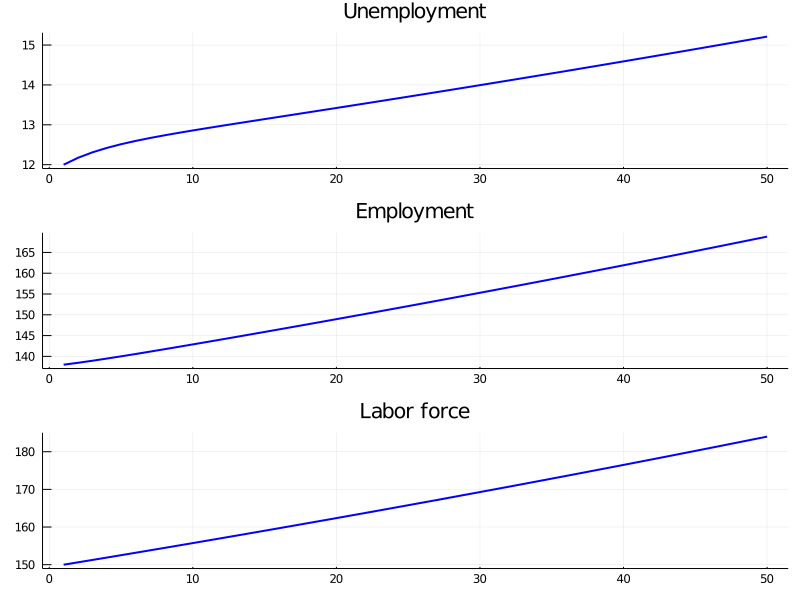The aggregates$ E_t $and$ U_t $don’t converge because their sum$ E_t + U_t $grows at rate$ g $. On the other hand, the vector of employment and unemployment rates$ x_t $can be in a steady state$ \bar x $if there exists an$ \bar x $such that •$ \bar x = \hat A \bar x $• the components satisfy$ \bar e + \bar u = 1 $This equation tells us that a steady state level$ \bar x $is an eigenvector of$ \hat A $associated with a unit eigenvalue. We also have$ x_t \to \bar x $as$ t \to \infty $provided that the remaining eigenvalue of$ \hat A $has modulus less that 1. This is the case for our default parameters: In : lm = LakeModel() A, Â = transition_matrices(lm) e, f = eigvals(Â) abs(e), abs(f)  Out: (0.6953067378358462, 1.0) Let’s look at the convergence of the unemployment and employment rate to steady state levels (dashed red line) In : lm = LakeModel() e_0 = 0.92 # initial employment rate u_0 = 1 - e_0 # initial unemployment rate T = 50 # simulation length xbar = rate_steady_state(lm) x_0 = [u_0; e_0] x_path = simulate_rate_path(lm, x_0, T) plt_unemp = plot(title ="Unemployment rate", 1:T, x_path[1, :],color = :blue, lw = 2, alpha = 0.5, grid = true, label = "") plot!(plt_unemp, [xbar], color=:red, linetype = :hline, linestyle = :dash, lw = 2, label = "") plt_emp = plot(title = "Employment rate", 1:T, x_path[2, :],color = :blue, lw = 2, alpha = 0.5, grid = true, label = "") plot!(plt_emp, [xbar], color=:red, linetype = :hline, linestyle = :dash, lw = 2, label = "") plot(plt_unemp, plt_emp, layout = (2, 1), size=(700,500))  Out: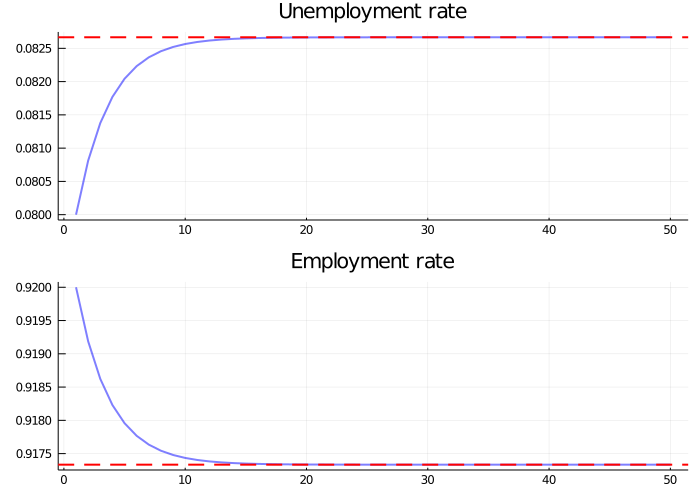## Dynamics of an Individual Worker¶ An individual worker’s employment dynamics are governed by a finite state Markov process. The worker can be in one of two states: •$ s_t=0 $means unemployed •$ s_t=1 $means employed Let’s start off under the assumption that$ b = d = 0 $. The associated transition matrix is then $$P = \left( \begin{matrix} 1 - \lambda & \lambda \\ \alpha & 1 - \alpha \end{matrix} \right)$$ Let$ \psi_t $denote the marginal distribution over employment / unemployment states for the worker at time$ t $. As usual, we regard it as a row vector. We know from an earlier discussion that$ \psi_t $follows the law of motion $$\psi_{t+1} = \psi_t P$$ We also know from the lecture on finite Markov chains that if$ \alpha \in (0, 1) $and$ \lambda \in (0, 1) $, then$ P $has a unique stationary distribution, denoted here by$ \psi^* $. The unique stationary distribution satisfies $$\psi^* = \frac{\alpha}{\alpha + \lambda}$$ Not surprisingly, probability mass on the unemployment state increases with the dismissal rate and falls with the job finding rate rate. ### Ergodicity¶ Let’s look at a typical lifetime of employment-unemployment spells. We want to compute the average amounts of time an infinitely lived worker would spend employed and unemployed. Let $$\bar s_{u,T} := \frac1{T} \sum_{t=1}^T \mathbb 1\{s_t = 0\}$$ and $$\bar s_{e,T} := \frac1{T} \sum_{t=1}^T \mathbb 1\{s_t = 1\}$$ (As usual,$ \mathbb 1\{Q\} = 1 $if statement$ Q $is true and 0 otherwise) These are the fraction of time a worker spends unemployed and employed, respectively, up until period$ T $. If$ \alpha \in (0, 1) $and$ \lambda \in (0, 1) $, then$ P $is ergodic, and hence we have $$\lim_{T \to \infty} \bar s_{u, T} = \psi^* \quad \text{and} \quad \lim_{T \to \infty} \bar s_{e, T} = \psi^*$$ with probability one. Inspection tells us that$ P $is exactly the transpose of$ \hat A $under the assumption$ b=d=0 $. Thus, the percentages of time that an infinitely lived worker spends employed and unemployed equal the fractions of workers employed and unemployed in the steady state distribution. ### Convergence rate¶ How long does it take for time series sample averages to converge to cross sectional averages? We can use QuantEcon.jl’s MarkovChain type to investigate this. Let’s plot the path of the sample averages over 5,000 periods In : using QuantEcon, Roots, Random  In : lm = LakeModel(d = 0, b = 0) T = 5000 # Simulation length @unpack α, λ = lm P = [(1 - λ) λ α (1 - α)]  Out: 2×2 Array{Float64,2}: 0.717 0.283 0.013 0.987 In : Random.seed!(42) mc = MarkovChain(P, [0; 1]) # 0=unemployed, 1=employed xbar = rate_steady_state(lm) s_path = simulate(mc, T; init=2) s̄_e = cumsum(s_path) ./ (1:T) s̄_u = 1 .- s̄_e s_bars = [s̄_u s̄_e] plt_unemp = plot(title = "Percent of time unemployed", 1:T, s_bars[:,1],color = :blue, lw = 2, alpha = 0.5, label = "", grid = true) plot!(plt_unemp, [xbar], linetype = :hline, linestyle = :dash, color=:red, lw = 2, label = "") plt_emp = plot(title = "Percent of time employed", 1:T, s_bars[:,2],color = :blue, lw = 2, alpha = 0.5, label = "", grid = true) plot!(plt_emp, [xbar], linetype = :hline, linestyle = :dash, color=:red, lw = 2, label = "") plot(plt_unemp, plt_emp, layout = (2, 1), size=(700,500))  Out: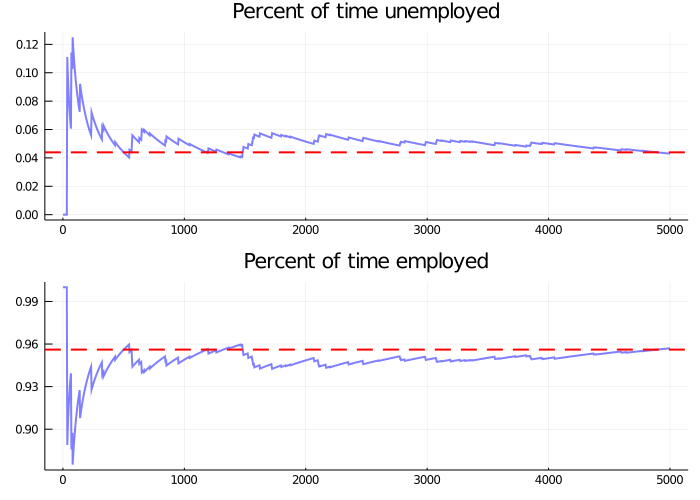The stationary probabilities are given by the dashed red line. In this case it takes much of the sample for these two objects to converge. This is largely due to the high persistence in the Markov chain. ## Endogenous Job Finding Rate¶ We now make the hiring rate endogenous. The transition rate from unemployment to employment will be determined by the McCall search model [McC70]. All details relevant to the following discussion can be found in our treatment of that model. ### Reservation Wage¶ The most important thing to remember about the model is that optimal decisions are characterized by a reservation wage$ \bar w $. • If the wage offer$ w $in hand is greater than or equal to$ \bar w $, then the worker accepts. • Otherwise, the worker rejects. As we saw in our discussion of the model, the reservation wage depends on the wage offer distribution and the parameters •$ \alpha $, the separation rate •$ \beta $, the discount factor •$ \gamma $, the offer arrival rate •$ c $, unemployment compensation ### Linking the McCall Search Model to the Lake Model¶ Suppose that all workers inside a lake model behave according to the McCall search model. The exogenous probability of leaving employment remains$ \alpha $. But their optimal decision rules determine the probability$ \lambda $of leaving unemployment. This is now $$\lambda = \gamma \mathbb P \{ w_t \geq \bar w\} = \gamma \sum_{w' \geq \bar w} p(w') \tag{1}$$ ### Fiscal Policy¶ We can use the McCall search version of the Lake Model to find an optimal level of unemployment insurance. We assume that the government sets unemployment compensation$ c $. The government imposes a lump sum tax$ \tau $sufficient to finance total unemployment payments. To attain a balanced budget at a steady state, taxes, the steady state unemployment rate$ u $, and the unemployment compensation rate must satisfy $$\tau = u c$$ The lump sum tax applies to everyone, including unemployed workers. Thus, the post-tax income of an employed worker with wage$ w $is$ w - \tau $. The post-tax income of an unemployed worker is$ c - \tau $. For each specification$ (c, \tau) $of government policy, we can solve for the worker’s optimal reservation wage. This determines$ \lambda $via (1) evaluated at post tax wages, which in turn determines a steady state unemployment rate$ u(c, \tau) $. For a given level of unemployment benefit$ c $, we can solve for a tax that balances the budget in the steady state $$\tau = u(c, \tau) c$$ To evaluate alternative government tax-unemployment compensation pairs, we require a welfare criterion. We use a steady state welfare criterion $$W := e \, {\mathbb E} [V \, | \, \text{employed}] + u \, U$$ where the notation$ V $and$ U $is as defined in the McCall search model lecture. The wage offer distribution will be a discretized version of the lognormal distribution$ LN(\log(20),1) $, as shown in the next figureWe take a period to be a month. We set$ b $and$ d $to match monthly birth and death rates, respectively, in the U.S. population •$ b = 0.0124 $•$ d = 0.00822 $Following [DFH06], we set$ \alpha $, the hazard rate of leaving employment, to •$ \alpha = 0.013 $### Fiscal Policy Code¶ We will make use of (with some tweaks) the code we wrote in the McCall model lecture, embedded below for convenience. In : function solve_mccall_model(mcm; U_iv = 1.0, V_iv = ones(length(mcm.w)), tol = 1e-5, iter = 2_000) @unpack α, β, σ, c, γ, w, E, u = mcm # necessary objects u_w = u.(w, σ) u_c = u(c, σ) # Bellman operator T. Fixed point is x* s.t. T(x*) = x* function T(x) V = x[1:end-1] U = x[end] [u_w + β * ((1 - α) * V .+ α * U); u_c + β * (1 - γ) * U + β * γ * E * max.(U, V)] end # value function iteration x_iv = [V_iv; U_iv] # initial x val xstar = fixedpoint(T, x_iv, iterations = iter, xtol = tol, m = 0).zero V = xstar[1:end-1] U = xstar[end] # compute the reservation wage w_barindex = searchsortedfirst(V .- U, 0.0) if w_barindex >= length(w) # if this is true, you never want to accept w̄ = Inf else w̄ = w[w_barindex] # otherwise, return the number end # return a NamedTuple, so we can select values by name return (V = V, U = U, w̄ = w̄) end  Out: solve_mccall_model (generic function with 1 method) And the McCall object In : # a default utility function u(c, σ) = c > 0 ? (c^(1 - σ) - 1) / (1 - σ) : -10e-6 # model constructor McCallModel = @with_kw (α = 0.2, β = 0.98, # discount rate γ = 0.7, c = 6.0, # unemployment compensation σ = 2.0, u = u, # utility function w = range(10, 20, length = 60), # wage values E = Expectation(BetaBinomial(59, 600, 400))) # distribution over wage values  Out: ##NamedTuple_kw#267 (generic function with 2 methods) Now let’s compute and plot welfare, employment, unemployment, and tax revenue as a function of the unemployment compensation rate In : # some global variables that will stay constant α = 0.013 α_q = (1 - (1 - α)^3) b_param = 0.0124 d_param = 0.00822 β = 0.98 γ = 1.0 σ = 2.0 # the default wage distribution: a discretized log normal log_wage_mean, wage_grid_size, max_wage = 20, 200, 170 w_vec = range(1e-3, max_wage, length = wage_grid_size + 1) logw_dist = Normal(log(log_wage_mean), 1) cdf_logw = cdf.(logw_dist, log.(w_vec)) pdf_logw = cdf_logw[2:end] - cdf_logw[1:end-1] p_vec = pdf_logw ./ sum(pdf_logw) w_vec = (w_vec[1:end-1] + w_vec[2:end]) / 2 E = expectation(Categorical(p_vec)) # expectation object function compute_optimal_quantities(c, τ) mcm = McCallModel(α = α_q, β = β, γ = γ, c = c - τ, # post-tax compensation σ = σ, w = w_vec .- τ, # post-tax wages E = E) # expectation operator @unpack V, U, w̄ = solve_mccall_model(mcm) indicator = wage -> wage > w̄ λ = γ * E * indicator.(w_vec .- τ) return w̄, λ, V, U end function compute_steady_state_quantities(c, τ) w̄, λ_param, V, U = compute_optimal_quantities(c, τ) # compute steady state employment and unemployment rates lm = LakeModel(λ = λ_param, α = α_q, b = b_param, d = d_param) x = rate_steady_state(lm) u_rate, e_rate = x # compute steady state welfare indicator(wage) = wage > w̄ indicator(wage) = wage > w̄ decisions = indicator.(w_vec .- τ) w = (E * (V .* decisions)) / (E * decisions) welfare = e_rate .* w + u_rate .* U return u_rate, e_rate, welfare end function find_balanced_budget_tax(c) function steady_state_budget(t) u_rate, e_rate, w = compute_steady_state_quantities(c, t) return t - u_rate * c end τ = find_zero(steady_state_budget, (0.0, 0.9c)) return τ end # levels of unemployment insurance we wish to study Nc = 60 c_vec = range(5, 140, length = Nc) tax_vec = zeros(Nc) unempl_vec = similar(tax_vec) empl_vec = similar(tax_vec) welfare_vec = similar(tax_vec) for i in 1:Nc t = find_balanced_budget_tax(c_vec[i]) u_rate, e_rate, welfare = compute_steady_state_quantities(c_vec[i], t) tax_vec[i] = t unempl_vec[i] = u_rate empl_vec[i] = e_rate welfare_vec[i] = welfare end plt_unemp = plot(title = "Unemployment", c_vec, unempl_vec, color = :blue, lw = 2, alpha=0.7, label = "",grid = true) plt_tax = plot(title = "Tax", c_vec, tax_vec, color = :blue, lw = 2, alpha=0.7, label = "", grid = true) plt_emp = plot(title = "Employment", c_vec, empl_vec, color = :blue, lw = 2, alpha=0.7, label = "", grid = true) plt_welf = plot(title = "Welfare", c_vec, welfare_vec, color = :blue, lw = 2, alpha=0.7, label = "", grid = true) plot(plt_unemp, plt_emp, plt_tax, plt_welf, layout = (2,2), size = (800, 700))  Out: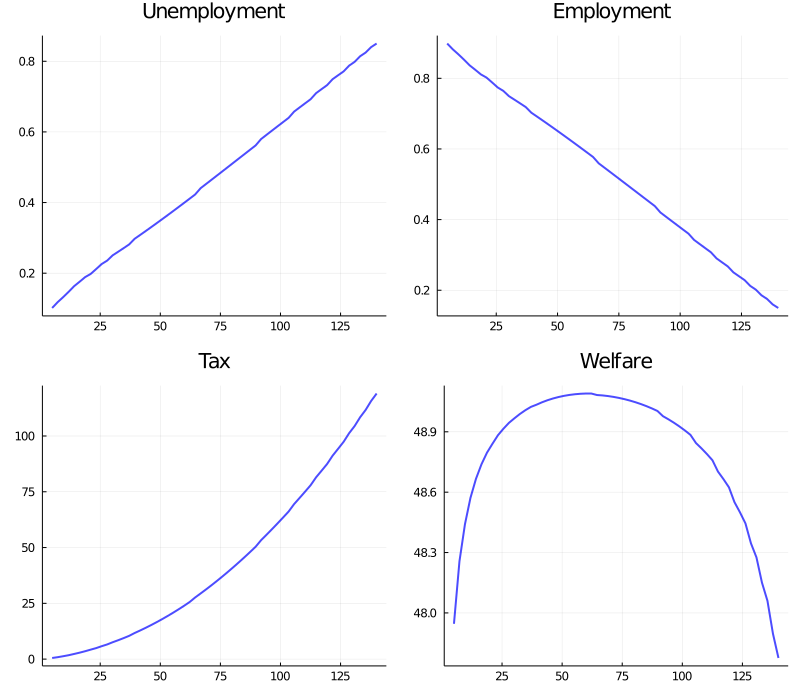Welfare first increases and then decreases as unemployment benefits rise. The level that maximizes steady state welfare is approximately 62. ## Exercises¶ ### Exercise 1¶ Consider an economy with initial stock of workers$ N_0 = 100 $at the steady state level of employment in the baseline parameterization •$ \alpha = 0.013 $•$ \lambda = 0.283 $•$ b = 0.0124 $•$ d = 0.00822 $(The values for$ \alpha $and$ \lambda $follow [DFH06]) Suppose that in response to new legislation the hiring rate reduces to$ \lambda = 0.2 $. Plot the transition dynamics of the unemployment and employment stocks for 50 periods. Plot the transition dynamics for the rates. How long does the economy take to converge to its new steady state? What is the new steady state level of employment? ### Exercise 2¶ Consider an economy with initial stock of workers$ N_0 = 100 $at the steady state level of employment in the baseline parameterization. Suppose that for 20 periods the birth rate was temporarily high ($ b = 0.0025 $) and then returned to its original level. Plot the transition dynamics of the unemployment and employment stocks for 50 periods. Plot the transition dynamics for the rates. How long does the economy take to return to its original steady state? ## Solutions¶ ### Exercise 1¶ We begin by constructing an object containing the default parameters and assigning the steady state values to x0 In : lm = LakeModel() x0 = rate_steady_state(lm) println("Initial Steady State:$x0")

Initial Steady State: [0.08266626766923271, 0.9173337323307673]


Initialize the simulation values

In :
N0 = 100
T = 50

Out:
50

New legislation changes $\lambda$ to $0.2$

In :
lm = LakeModel(λ = 0.2)

Out:
(λ = 0.2, α = 0.013, b = 0.0124, d = 0.00822)
In :
xbar = rate_steady_state(lm) # new steady state
X_path = simulate_stock_path(lm, x0 * N0, T)
x_path = simulate_rate_path(lm, x0, T)
println("New Steady State: $xbar")  New Steady State: [0.11309294549489424, 0.8869070545051054]  Now plot stocks In : x1 = X_path[1, :] x2 = X_path[2, :] x3 = dropdims(sum(X_path, dims = 1), dims = 1) plt_unemp = plot(title = "Unemployment", 1:T, x1, color = :blue, grid = true, label = "", bg_inside = :lightgrey) plt_emp = plot(title = "Employment", 1:T, x2, color = :blue, grid = true, label = "", bg_inside = :lightgrey) plt_labor = plot(title = "Labor force", 1:T, x3, color = :blue, grid = true, label = "", bg_inside = :lightgrey) plot(plt_unemp, plt_emp, plt_labor, layout = (3, 1), size = (800, 600))  Out: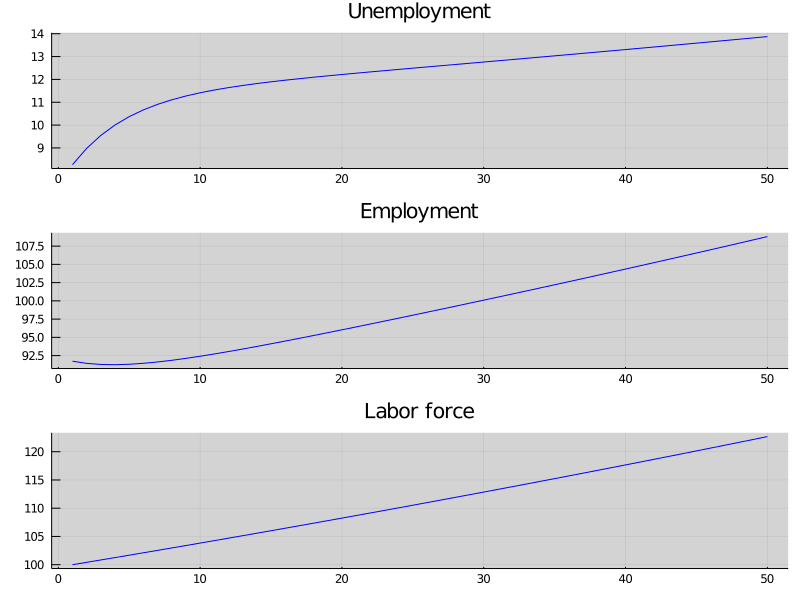And how the rates evolve In : plt_unemp = plot(title = "Unemployment rate", 1:T, x_path[1,:], color = :blue, grid = true, label = "", bg_inside = :lightgrey) plot!(plt_unemp, [xbar], linetype = :hline, linestyle = :dash, color =:red, label = "") plt_emp = plot(title = "Employment rate", 1:T, x_path[2,:], color = :blue, grid = true, label = "", bg_inside = :lightgrey) plot!(plt_emp, [xbar], linetype = :hline, linestyle = :dash, color =:red, label = "") plot(plt_unemp, plt_emp, layout = (2, 1), size = (800, 600))  Out: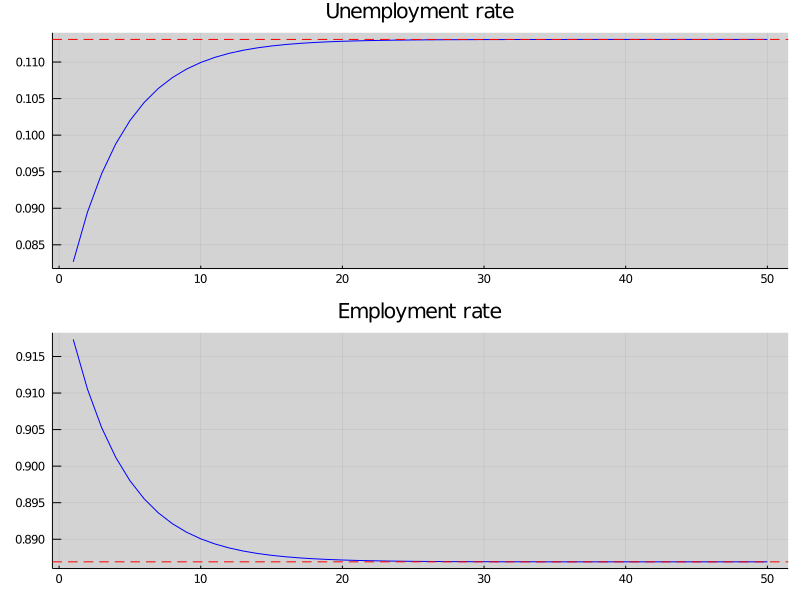We see that it takes 20 periods for the economy to converge to it’s new steady state levels. ### Exercise 2¶ This next exercise has the economy experiencing a boom in entrances to the labor market and then later returning to the original levels. For 20 periods the economy has a new entry rate into the labor market. Let’s start off at the baseline parameterization and record the steady state In : lm = LakeModel() x0 = rate_steady_state(lm)  Out: 2-element Array{Float64,1}: 0.08266626766923271 0.9173337323307673 Here are the other parameters: In : b̂ = 0.003 T̂ = 20  Out: 20 Let’s increase$ b $to the new value and simulate for 20 periods In : lm = LakeModel(b=b̂) X_path1 = simulate_stock_path(lm, x0 * N0, T̂) # simulate stocks x_path1 = simulate_rate_path(lm, x0, T̂) # simulate rates  Out: 2×20 Array{Float64,2}: 0.0826663 0.0739981 0.0679141 … 0.0536612 0.0536401 0.0536253 0.917334 0.926002 0.932086 0.946339 0.94636 0.946375 Now we reset$ b \$ to the original value and then, using the state after 20 periods for the new initial conditions, we simulate for the additional 30 periods

In :
lm = LakeModel(b = 0.0124)
X_path2 = simulate_stock_path(lm, X_path1[:, end-1], T-T̂+1)    # simulate stocks
x_path2 = simulate_rate_path(lm, x_path1[:, end-1], T-T̂+1)     # simulate rates

Out:
2×31 Array{Float64,2}:
0.0536401  0.0624842  0.0686335  …  0.0826652  0.0826655  0.0826657
0.94636    0.937516   0.931366      0.917335   0.917335   0.917334

Finally we combine these two paths and plot

In :
x_path = hcat(x_path1, x_path2[:, 2:end])  # note [2:] to avoid doubling period 20
X_path = hcat(X_path1, X_path2[:, 2:end])

Out:
2×50 Array{Float64,2}:
8.26663   7.36118   6.72069   6.26524  …   8.45538   8.49076   8.52628
91.7334   92.1168   92.238    92.1769      93.8293   94.2215   94.6153
In :
x1 = X_path[1,:]
x2 = X_path[2,:]
x3 = dropdims(sum(X_path, dims = 1), dims = 1)

plt_unemp = plot(title = "Unemployment", 1:T, x1, color = :blue, lw = 2, alpha = 0.7,
grid = true, label = "", bg_inside = :lightgrey)
plot!(plt_unemp, ylims = extrema(x1) .+ (-1, 1))

plt_emp = plot(title = "Employment", 1:T, x2, color = :blue, lw = 2, alpha = 0.7, grid = true,
label = "", bg_inside = :lightgrey)
plot!(plt_emp, ylims = extrema(x2) .+ (-1, 1))

plt_labor = plot(title = "Labor force", 1:T, x3, color = :blue, alpha = 0.7, grid = true,
label = "", bg_inside = :lightgrey)
plot!(plt_labor, ylims = extrema(x3) .+ (-1, 1))
plot(plt_unemp, plt_emp, plt_labor, layout = (3, 1), size = (800, 600))

Out: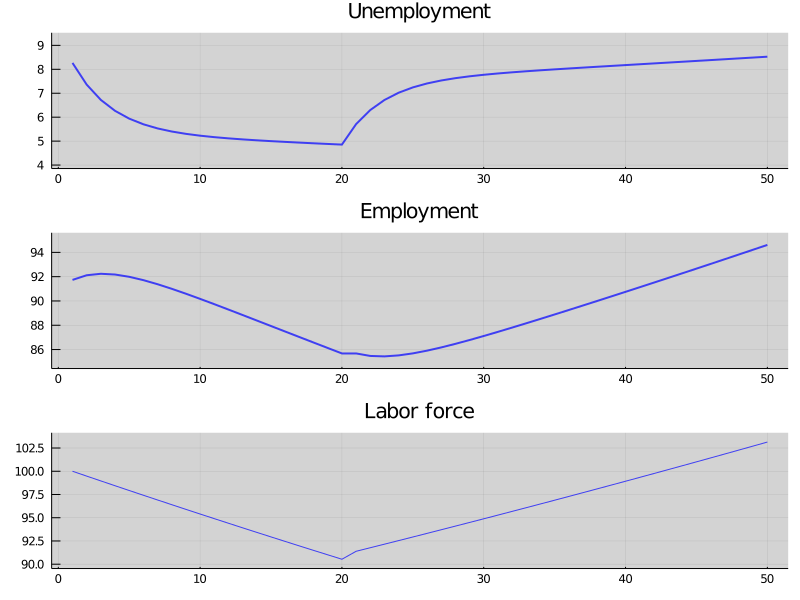And the rates

In :
plt_unemp = plot(title = "Unemployment Rate", 1:T, x_path[1,:], color = :blue, grid = true,
label = "", bg_inside = :lightgrey, lw = 2)
plot!(plt_unemp, [x0], linetype = :hline, linestyle = :dash, color =:red, label = "", lw = 2)

plt_emp = plot(title = "Employment Rate", 1:T, x_path[2,:], color = :blue, grid = true,
label = "", bg_inside = :lightgrey, lw = 2)
plot!(plt_emp, [x0], linetype = :hline, linestyle = :dash, color =:red, label = "", lw = 2)

plot(plt_unemp, plt_emp, layout = (2, 1), size = (800, 600))

Out: